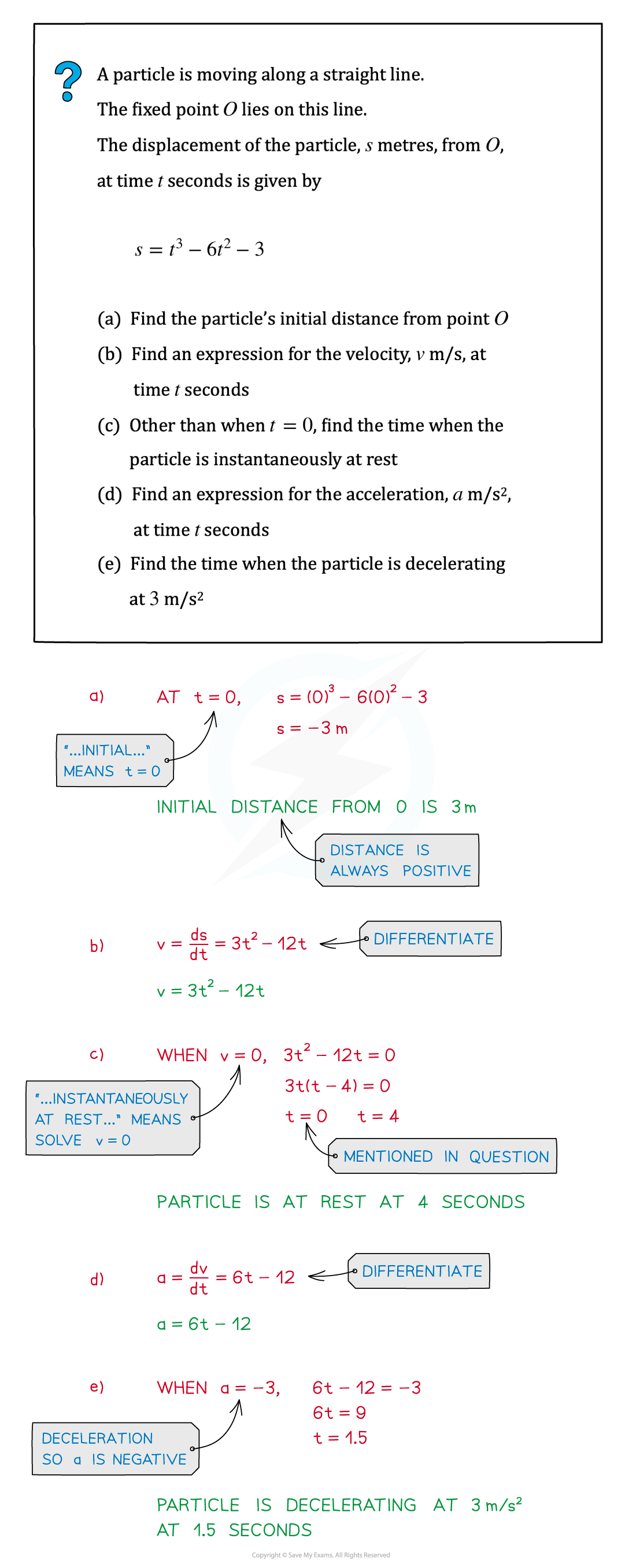# Edexcel IGCSE Maths 复习笔记 3.11.4 Differentiation - Kinematics

Edexcel IGCSE Maths 复习笔记 3.11.4 Differentiation - Kinematics

#### What is kinematics?

• Kinematics is the analysis of the motion of a particle linking the three vector quantities displacementvelocity and acceleration – see below
• Motion is in a straight line – think of the particle as moving along a number line
• The number line has a fixed point O (the origin)
• The number line has both negative and positive values
• The particle can move in both directions along the number line

• Note that in kinematics, a particle is an object – it could be a football, a car, a train - anything that has motion.  A particle is modelled as taking up a single point in space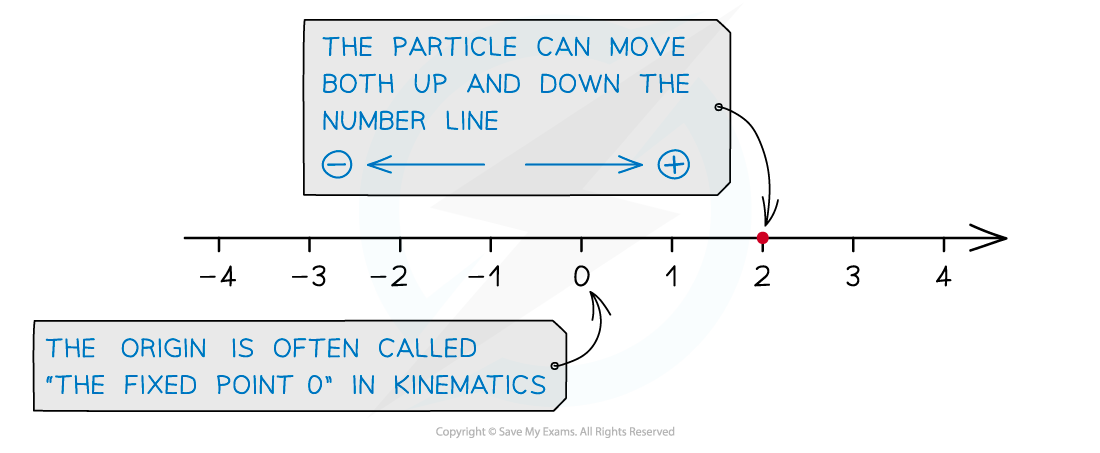• Ensure you are familiar with Differentiation - Basics andDifferentiation – Turning Points before continuing
• It may be wise to look at Differentiation – Problem Solving too

#### What is displacement; isn’t it the same as distance?

• Displacement is a vector quantity, so it can be negative
• Distance is always positive

• Displacement is measured from the fixed point O
• The letter s is used for displacement
• It is usually measured in metres (m)

• If s = 4 then the distance from the origin is 4 m and the particle is 4 m “in front of” the origin
• If s =-5 then the distance from the origin is 5 m and the particle is 5 m “behind” the origin
• The + or - indicates the particle’s position relative to the origin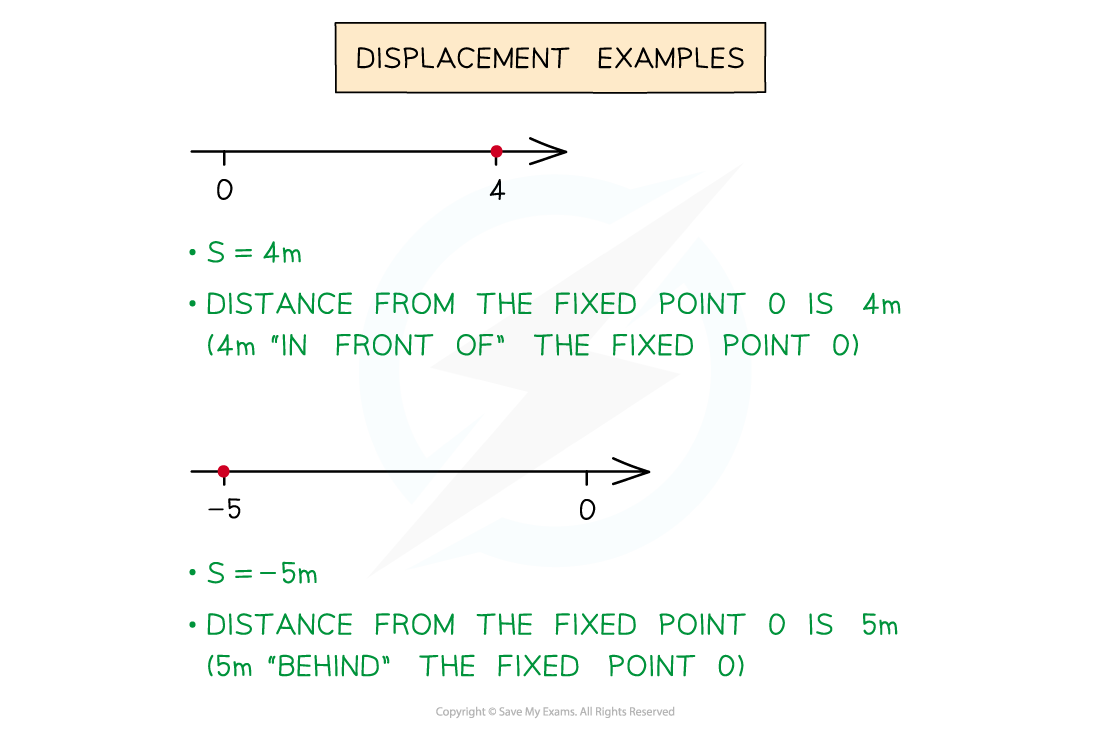• Displacement is a function of time, t, where time is usually measured in seconds
• eg. s = 3t3 - 2t + 1

At time t = 0s = 1At time t = 2s = 21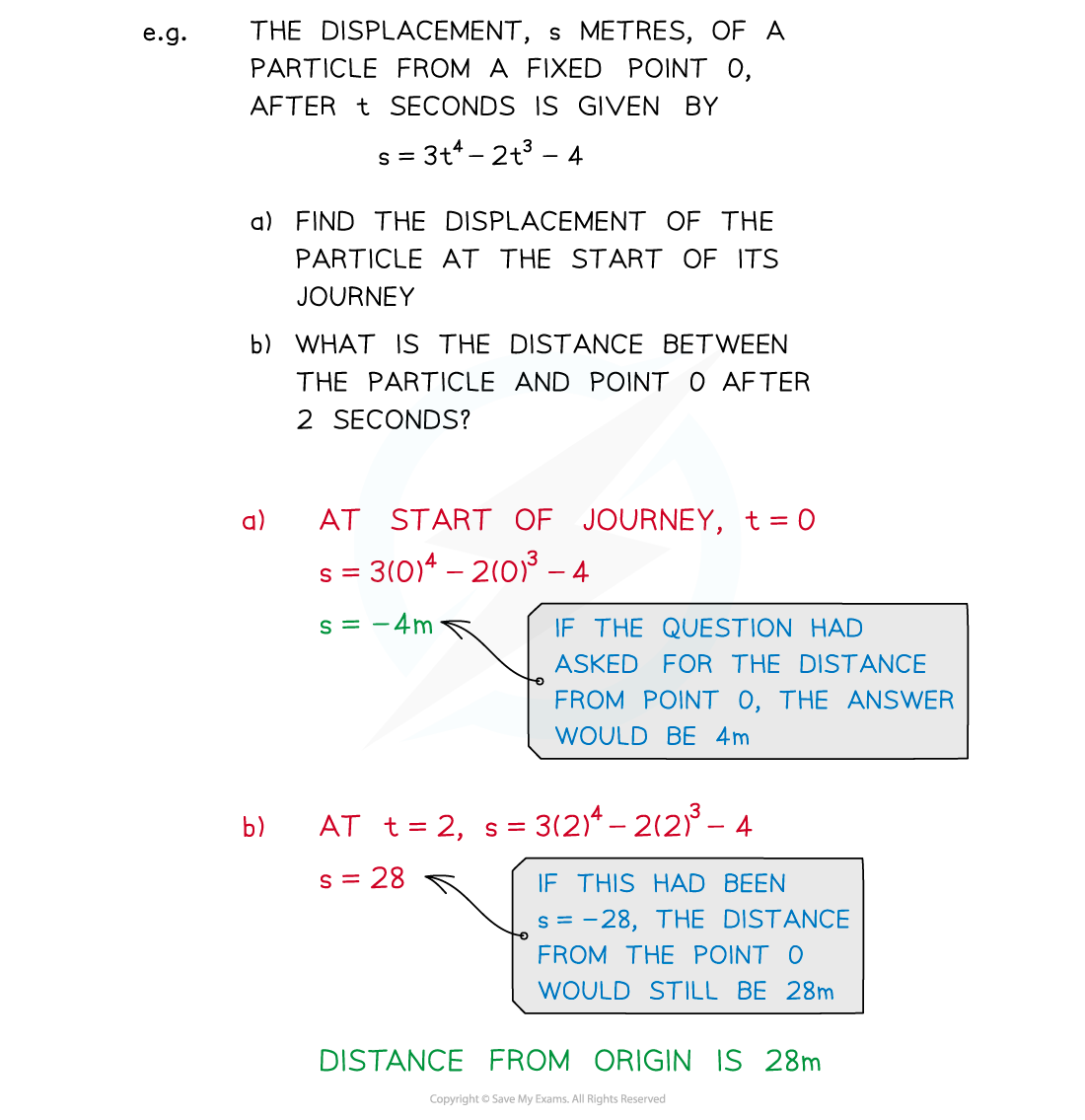#### What is velocity; isn’t it the same as speed?

• Velocity is a vector quantity, so it can be negative
• Speed is always positive

• The letter v is used for velocity
• It is usually measured in metres per second (m/s)

• If v = 3 then the speed of the particle is 3 m/s and it is moving in the positive direction
• If v = -6 then the speed of the particle is 5 m/s and it is moving in the negative direction
• The + or - indicates the particle’s direction of motion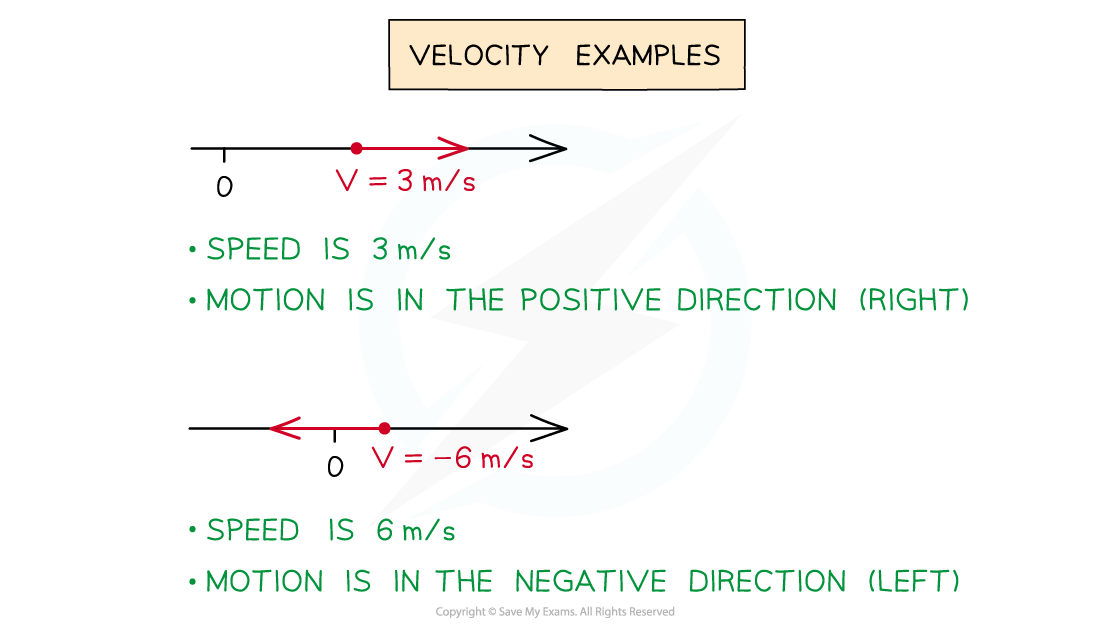• Velocity is a function of timet, and is the rate of change of displacement
• To find vdifferentiate s, ie. v = ds/dt

If s = t3 - 2t2then v = ds/dt = 3t2 – 4t

• If velocity is zero then the particle is stationary (not moving)#### What is acceleration?

• Acceleration is a vector quantity, so it can be negative
• The magnitude of acceleration is always positive

• The letter a is used for acceleration
• It is usually measured in metres per square second (m/s2)

• If a = 1 then the magnitude of acceleration is 1 m/s2 and the particle is accelerating (velocity increasing)
• If a = -6 then the magnitude of acceleration is 6 m/s2 and the particle is decelerating (velocity decreasing)
• The + or - indicates whether the particle is accelerating or decelerating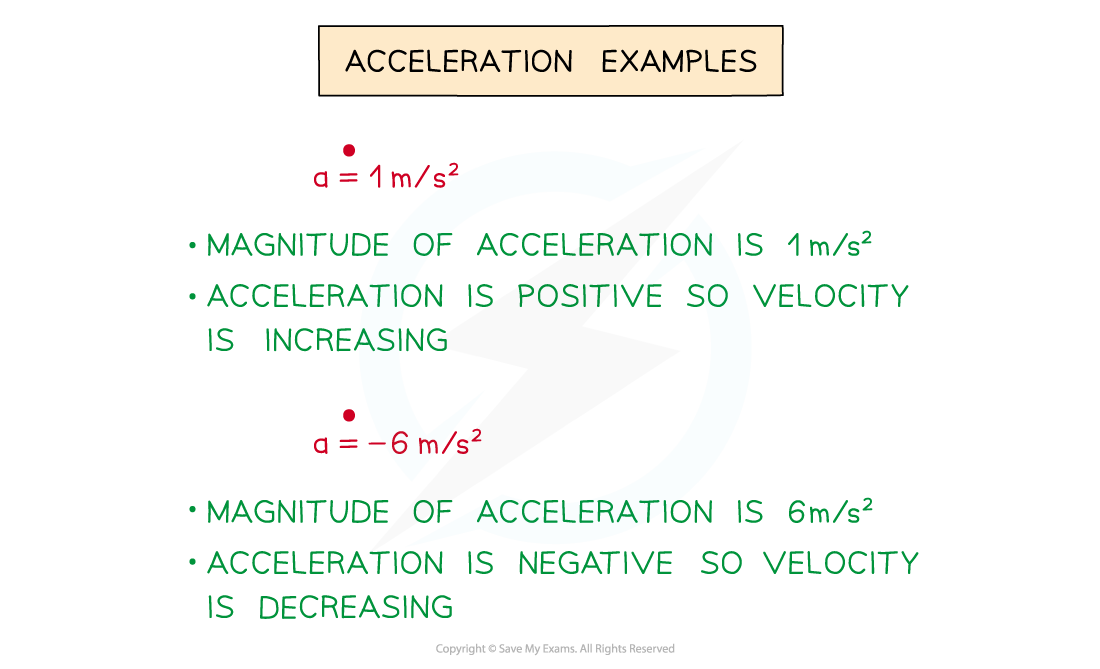• Acceleration is a function of timet, and is the rate of change of velocity
• To find a, differentiate v, ie. a = dv/dt

If v = 3t2 – 4tthen a = dv/dt = 6t - 4

• If acceleration is zero then the particle is moving at a constant velocity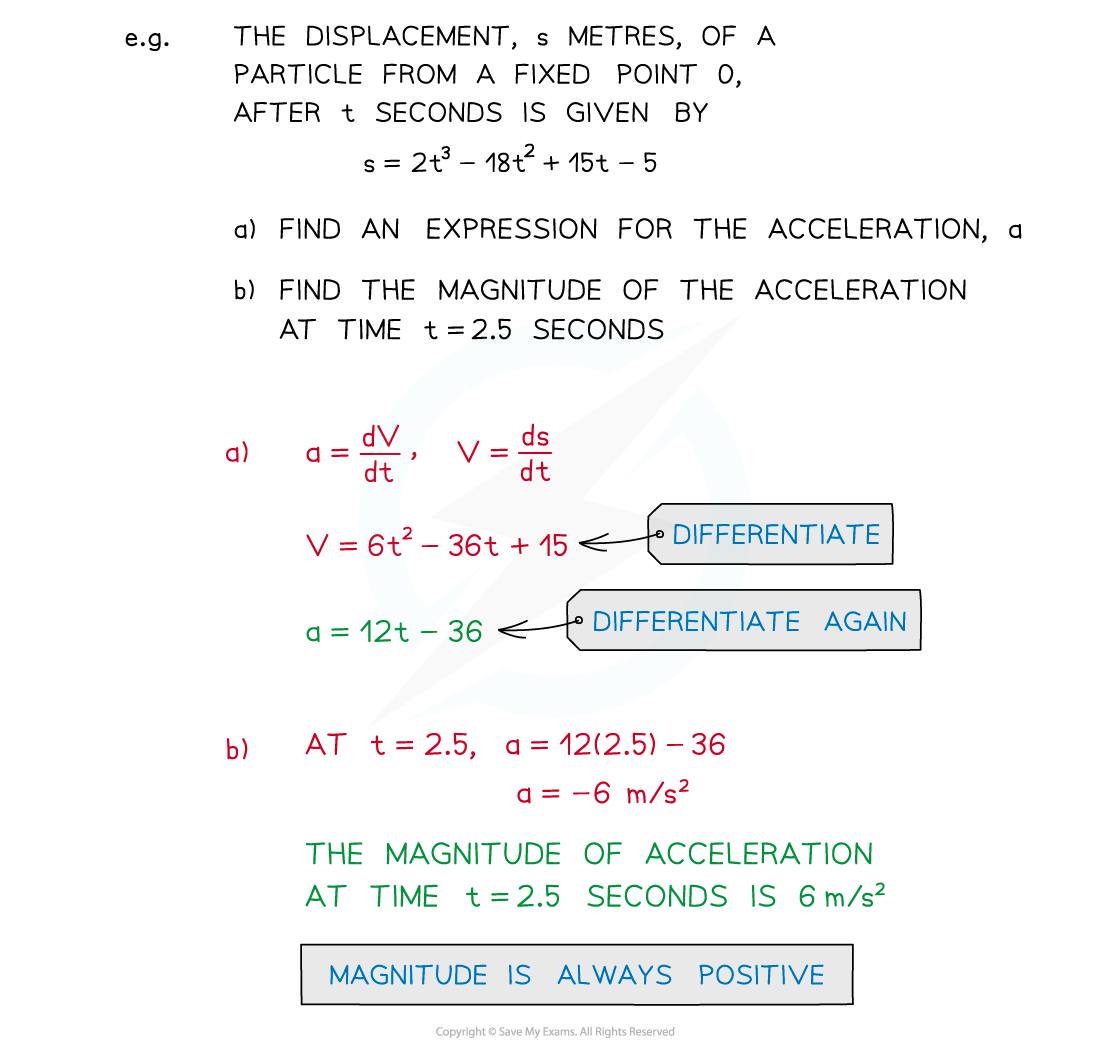#### How do I solve kinematics problems?

• Be clear about how the three quantities are related through differentiation
• v = ds/dt
• a = dv/dt• There are some key phrases to look out for
• “... initial ...” / “... initially ...”

This means at the start, so when t = 0

• “... at rest ...”

This means the particle is stationaryso v = 0

• “... instantaneously ...”This means at some point in time, for some value of t

• For example,“Find the value(s)s of t for which the particle is instantaneously at rest”
• means find the time(s) when v = 0,
• ie. solve the equation v = 0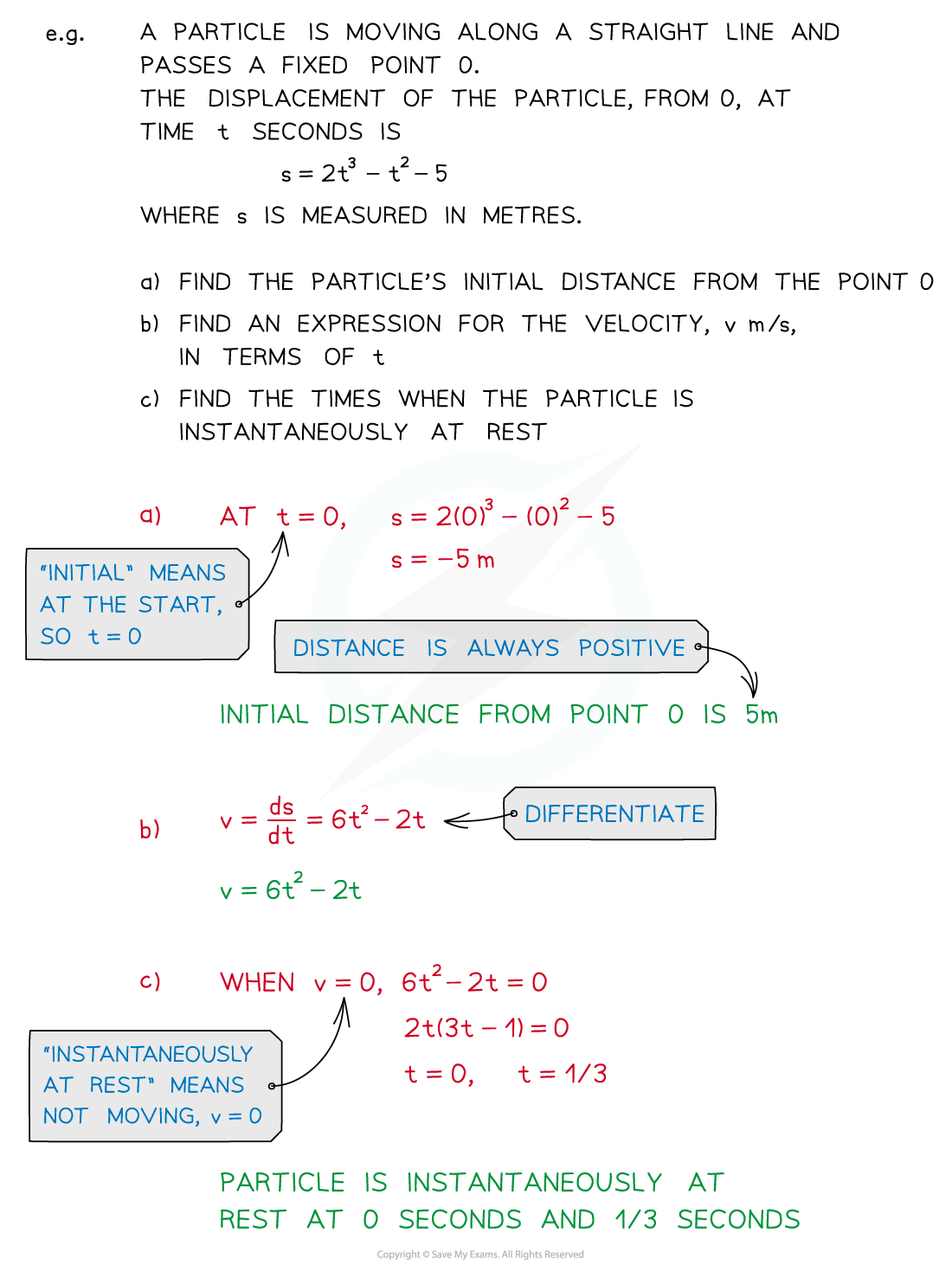#### Exam Tip

Displacementvelocity and acceleration can all be negative whereas distancespeed and magnitude of acceleration are always positive.

#### Worked Example